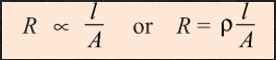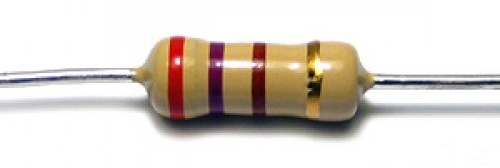In the previous article (Basics of voltage & current), we have seen that the force that provokes electrons is called as voltage, and the flow of electron in a closed circuit is called as current.

Now, the question is when the current flows through this closed circuit is there any opposition experience by that current? And is there any relationship between voltage and current?

• what is Resistance?
• Parameters on which resistance depends
• what is positive and negative temperature coefficient?
• Resistance in series and parallel
• Ohm’s law with the practical

RESISTANCE:

There are some materials in which electrons are very tightly bounded, and because of that that they do not allow electrons to go through them, or simply you can say that they offer some opposition to the flow of electron. And there are also some kind of materials which the electrons are loosely bounded and hence they allow the flow of electrons through them, but that flow of electron still experience some kind of opposition and this opposition of offered by a conductor to the flow of electron is nothing but called as RESISTANCE. The name resistance is a given because the conductor try to resist the flow of electron through them.

Material which has electron very tightly bounded is called as bad conductor of electricity, because they have very high resistance and because of that the electricity cannot flow through them.

And the material which has electron very loosely bounded they are called as good conductor of electricity because they offer a very low resistance to the flow of electricity through them.

So, simply you can say that a resistance is the property of a conductor which resist the flow of electron through them.

Parameters on which resistance depends:

The resistance of a conductor depends upon following main four parameters,

1. Length of the conductor
2. Cross section area
3. Material used
4. Temperature

Resistance is directly proportional to the length of the conductor that means resistance will increase with the increase in length of the conductor, and it will decrease with decrease in the length of the conductor.The resistance is inversely proportional to the cross-sectional area of the conductor.  Most of the students don’t understand what cross section area is?  so, you can say that if you are having a conductor as shown belowand if you saw that conductor from the front side it will look like thisAnd this is nothing but called as your cross-section area. So, with the increase in cross sectional area your resistance is going to decrease, and vice versa

The Material used highly affects the resistance. For example, if you use Steel you will get more resistance, and if you use copper resistance will be lesser. So, the type of material really makes difference.

The fourth and important parameter is temperature. With the increase in temperature the resistance of some material increases. Now, the question is how temperature affects the resistance of a material?  The answer is, with the increasing in temperature your material’s length will expands or get it will get quench, that depend upon the type of material, for example say with the increase in temperature your material got expand so, because of that expansion the resistance will increase.  This increase in resistance with increase in temperature generally takes place in conductor, and the material in which this happens is called to have positive temperature coefficient. Why positive temperature coefficient?  because the material responded to increase in temperature with the increase in resistance, or simply we can say that it responded in the positive way, and that is why it is called as positive temperature coefficient.

Now, sometimes with the increase in temperature the resistance decreases, and the material in which this happens is called to have negative temperature coefficient. now why negative temperature coefficient?  because your material responded to the increase in temperature with decrease in resistance or simply in the negative way and that is why it is called as negative temperature coefficient. This generally happens in insulators.

So, from the discussion up till now, we can make a simple formula for resistance and that is,Where,

• R = Resistance
• l = length of the conductor
• A = Area of cross – section
• p = resistivity of the material used

Unit of the resistance is OHM.

RESISTANCE IN SERIES & PARALLEL:

With this resistance, we can do some magic tricks yes, I may sound crazy, but I call it a magic trick.

Let’s say you are having two resistance like you are seeing in the following picture. This type of resistance can be added in the circuit to increase the total resistance they have some specific amount of resistance in them like for example, 1 ohms, 2 ohms, 5 ohms etc.Let’s say you are having two such 5 ohms resistance, But you want a 10 ohm resistance, so what you will do you will simply connect the two resistance in series as shown in the following figure and bingo,  you will have 10 ohm resistance.So, the conclusion of this simple magic trick is Resistance adds up in series.

Total Resistance = R1+R2

Now, some people can say I want resistance less than 5 ohms, well, no worries we have a magic trick for that too, simply connect the two resistance in parallel and you will have resistance less than 5 Ohm, that’s all you got that.So, the conclusion of our small magic trick is, Resistance reduces in parallel.

Total Resistance in Parallel: 1/R1 + 1/R2

So, the overall conclusion of Resistance part is,

• Resistance is a property of a material which opposes the flow of electrons through them.
• Resistance depends upon length of the material, cross sectional area, material used, and temperature.
• Material in which resistance increases with the temperature is called to have positive temperature coefficient.
• Material in which resistance decreases with increase in temperature is called to have negative temperature coefficient.
• Resistance add up in series.
• Resistance reduces in parallel.

OHM’S LAW:

We should be thankful to Mr. ohms, because he found out the Ohm’s law, which made the life of an electrical engineer easy.

Ohm’s law has a number of practical applications.  like the law can be used to calculate current flowing through the system, or the voltage applied across a circuit,  or the resistance of the circuit and it can also be used to calculate the overall power of the circuit and many more application.

So, let’s see what Ohm’s law states us,

It says that, current flowing through a closed circuit is directly proportional to the voltage applied across it provided that resistance, temperature and type of material remains same.

In simple words, Mr. ohm told that with the increase in voltage your current will increase, and with decrease in voltage your current will decrease but, yes there is a “but”, the resistance and the parameter which affects the resistance should remain the same.

So, from this statement we can make a formula,

V = IR

And with the help of this formula we can calculate any one unknown value for e.g. voltage, current or resistance.

I = V/R

R = V/I

When students are asked about the Ohm’s law, they say that Ohm’s law is V=IR.

But you have to always remember that whenever you are asked about the ohm’s law you have to tell them the “statement”, not the formula alone, because the formula depend upon that statement only.

Now we will do a practical which will prove the Ohm’s law.

To do that we have a load that is, a 12-volt dc fan.  In first case, we will apply a 9 volt to that fan and we will record the current flowing through that fan.so, as you can see in the above image the current comes out to be 0.06 A.

Now in second case we will increase the voltage to 12 volts and we’ll keep the resistance constant.  Now as per as the Ohm’s law is concern the current should increase with increase in voltage provided with the constant resistance. So, we will check whether our current increases with 12 volts or not.so, as you can see from the above image with the increase in voltage or current has also been increased to 0.11 A.

Now we will check what resistance makes the change. So, to do that we will add some resistance in series with our 12 volts supply and now we will calculate the current flowing through the fan,so, as you can see from the above image with the increase in the resistance our current has been reduced to 0.09 A.

So, this practical proves the Ohm’s law, and it also shows the effect of the resistance on the current.

So, the overall conclusion of the Ohm’s law is

• Current increases with the increase in voltage provided that resistance should remain constant
• V=IR
• Current is inversely proportional to the resistance that means with the increase in resistance your current will decrease and vice versa.

You can also watch a video on resistance and the Ohm’s law in which you will be able to see the practical, just click on the following link,

Resistance and Ohm’s law (Video)

(225)

# Resistance & Ohm’s Law | Easiest Explanation (Article)

| By Reading, The Basics |